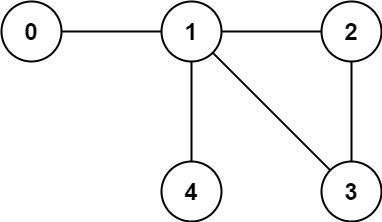# 261. Graph Valid Tree

Graph Valid Tree - LeetCode You have a graph of n nodes labeled from 0 to n - 1. You are given an integer n and a list of edges where edges[i] = [ai, bi] indicates that there is an undirected edge between nodes ai and bi in the graph. Return true if the edges of the given graph make up a valid tree, and false otherwise.

Example 1:Input: n = 5, edges = [[0,1],[0,2],[0,3],[1,4]] Output: true Example 2:Input: n = 5, edges = [[0,1],[1,2],[2,3],[1,3],[1,4]] Output: false C:

• code dfs
``````class Solution:
def validTree(self, n: int, edges: List[List[int]]) -> bool:

if len(edges) != n - 1: return False

adj_list = [[] for _ in range(n)]
for A, B in edges:

# We still need a seen set to prevent our code from infinite
# looping if there *is* cycles (and on the trivial cycles!)
seen = {0}
stack = 

while stack:
node = stack.pop()
if neighbour in seen:
continue
stack.append(neighbour)

return len(seen) == n
``````
• code dsu
``````class Solution:
def validTree(self, n: int, edges: List[List[int]]) -> bool:
if len(edges) != n-1: return False
root = [i for i in range(n)]
rank =  * n
def find(i):
if i == root[i]:
return i
root[i] = find(root[i])
return root[i]

def union(i, j):
rti = find(i)
rtj = find(j)
if rti == rtj:
return False # cycle found
if rank[rti] < rank[rtj]:
root[rti] = rtj
elif rank[rti] > rank[rtj]:
root[rtj] = rti
else:
root[rtj] = rti
rank[rti] += 1
return True

for i,j in edges:
if not union(i, j):
return False

return True
``````